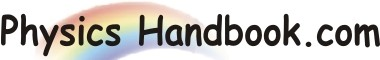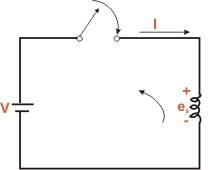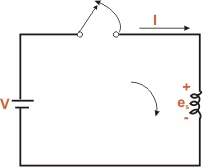HOME TOPICS DEFINITIONS TABLES LAWS INVENTIONS EXPERIMENTS QUIZ VIDEOS
 A B C D E F G H I J K L M N O P Q R S T U V W X Y Z
Self - Induction

When current in a coil increases or decreases, there is a change in magnetic flux linking the coil. Hence, an e.m.f. is induced in the coil. This is called self - induced e.m.f. (es) and the process is it opposes the cause the has produced it. Now the causes of this induced e.m.f. is the change in magnetic flux through the coil (i.e. change of current in the coil). Hence, the induced e.m.f. will oppose the change of current in the coil. If current in the coil is decreasing the induced e.m.f. will oppose the decrees in current.

The phenomenon of e.m.f. in a thermocouple when its two junction are at different temperature is known as Seebeck Effect.Fig (a)
Illustration:

Figure illustrates the phenomenon of self-induction. In Fig (a), closing the switch is an attempt to increase the current in the coil (i.e. to change it from zero to some positive value). As a result, e.m.f. esis induced in the coil in such a direction to oppose the increase in current i.e. induced e.m.f. acts in a direction opposite to that of applied voltage V. In Fig (b),the opening of switch is an attempt or reduce the current in the coil (i.e. to change it from some positive value to zero). Again e.m.f.esis induced in the direction of the applied voltage V.Fig (b)
It may be noted that the e.m.f. induced in a coil will persist so long as the current in the coil is changing. Thus in the above two cases, the induced voltage eventually reduces to zero because there is no continuous attempt to change the current beyond the instant that the switch is closed or opened.